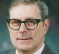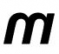## LTE IFFT Sampling and Nyquist RateStarted by 3 years ago6 replieslatest reply 3 years ago2888 views

Hello,

I started to work as a developer in LTE scheduling area. I was reading OFDM, IFFT procedures, my knowledge of signal processing is not that good and confused with Sampling Rate used in LTE.

For a 20MHz LTE system, the used Bandwidth for 1200SCs is 18MHz which means that minimum fs (sampling frequency) must be >= 36Mhz. But in all resources about LTE physical layer, it is stated that sampling rate is 30.72Mhz = 2048 samples in 66.67micro-sec. My understanding is that, this is sampling rate given in the frequency domain based on real (positive) frequency range of DFT samples (<fs/2). So we have 1200 samples from 1200Subcarriers and 848 zeropadding which gives 2048 DFT samples in the positive frequency range. When we take IFFT, I guess we also generate 2048 samples in the negative frequency range (which are just complex conjugates) so in total it makes 4096 IFFT samples and taking IFFT will give 4096 time domain samples in 66.67micro-sec which corresponds to actual time domaing sampling rate of 61.44MHz. Nyquist rate is 61.44MHz/2 = 30.72MHz which is > 18MHz of LTE used spectrum.

Do I understand correctly ? Is there any references describing full IFFT process in detail for LTE ?

Thanks.

[ - ]Zack, I'm not familiar with the application and didn't even know what the initials LTE stood for. (Side comment - the first time you use a a letter sequence abbreviation, you usually should give the full name, even if it is something you think should be instinctively known, like FFT or DSP.)

It says that to reproduce a signal with bandwidth B, it suffices to have B samples per second. It does not say how you get those samples. Commonly, the signal is available to sample and equally spaced samples are OK if the signal is centered on zero frequency.

In your case, the signal is probably embedded in a sea of other signals, plus, of course, noise. To get the needed samples of the desired signal by sampling you probably begin by some sort of analog filtering process to remove other signals. If you are willing to use a higher sampling rate that the B samples per second required to reproduce the signal, you can have some other signals present as long as they don't end up in the same band as the desired signal after sampling, due to aliasing.

You can also allow other signals in the same band if your aim is not to reproduce the signal but to extract information from it. That would require you to know that those other signals wouldn't interfere with the information you want to extract. As an example, if the desired signal has encoding with redundancy, you wouldn't need to clean out other signals of very low energy, and if the signals are mutually encoded to be orthogonal they could be in the same frequency band at the same time.

I doubt that this has been helpful.  But I once had an extreme example where I needed to recover the waveshape of a periodically repeating signal when I didn't know its frequency and I was restricted to using equally spaced samples provided to me, but with many periods of the periodic waveform between successive samples. The bandwidth of a periodic signal of unknown frequency is the uncertainty of its first harmonic, and by that measure I had far too few samples per second. So I couldn't recover the signal but I could still recover its waveshape (in the sense of plotting the waveform on a rubber sheet and not knowing by how much it was stretched, or even if it was time reversed).

[ - ]"For a 20MHz LTE system, the used Bandwidth for 1200SCs is 18MHz which means that minimum fs (sampling frequency) must be >= 36Mhz."

No, the 18MHz is the bandwidth of a complex signal (spectrum is not symmetrical). Nyquist criteria in this case translates to a required sampling rate of complex signals of at least 18 MHz (which you could say is having the two components of the complex signal (I and Q) sampled each at 18MHz which you could consider an effective sampling rate of 36MHz (the effective amount of "real" data). By adding the guard subcarriers to reach a power- of-two number of samples, we get 30.72 MHz = 2048*15kHz. Again we're talking about a complex FFT of size 2048 which means processing 4096 "numbers".

Anyway, the important thing for you to realize is that we're talking about complex signals, so the negative frequencies are not "just complex conjugates". The 4096 IFFT samples are really 2x2048 samples coming out of a 2048 complex IFFT engine, and are fed to two parallel DACs (or a quadrature DAC) each running at 30.72MHz. This is not the same thing as a 4096 IFFT running at 61.44MHz, but the "dimensionality" of Nyqusit holds however you put it.

[ - ]To be more specific the orthogonality requirement is more restrictive than sampling rule for ofdm based processing.

Orthogonality requires symbol time to be inverse of subcarrier spacing.

The number of subcarriers chosen and spacing is a matter of protocol, the size of fft should be power of 2(for implementation sake, except for 15MHz LTE) but more than the number of subcarriers.  i.e. 2048 for this case. The spacing chosen is 15KHz (4G) or multiples of that(5G). To centre bins 15KHz apart the sampling rate need to be 2048*15KHz as then fft bin resolution becomes 30.72/2048.

The 1200 subcarriers chosen is what 4G decided but 5G can go further to more than 1200 then the restriction moves to DUC filtering.

Sampling rule is less restrictive here as the edge of band is at 600/2048 * 30.72 = 9Mhz, hence 30.72 is more than enough.

[ - ]your understanding needs plenty polishing regarding I/Q and baseband.

20MHz LTE generation at eNB: 1200 subcarriers (frequency domain data as I/Q) meant to go through ifft of 2k to get 2048 I/Q time domain samples then cp added. Then sampled at 30.72Msps definitely. This is important for orthogonality.

[ - ]Hi

LTE signal is sampled at 30.72 MHz in time domain. As we use complex baseband signal, is means your whole system bandwidth might be 30.72 MHz exactly.

As we produce time domain signal with help of iFFT, one may think signal frequency composition is defined by iFFT frequency inputs. There are 2048 total frequency inputs to iFFT block. Unused DC carrier falls onto the middle, then you have 1023 bins to the right, -1024 bins to the left. Now see, sub-carrier spacing is 15 kHz, multiply by 1024 carriers to the left, it yields 15kHz*1024=15.36MHz, that's to the left only. And there are (almost) same 15.36MHz to the right, which gives total system bandwidth of 30.72 MHz.

Next about 18/20 MHz bandwidth. It happens, because we don't use all 2048 frequency inputs. Instead, we use only 1200 of them and set to zero remaining inputs. These 1200 frequency inputs are centered around DC, so again 15kHz*600=9MHz to each side, total 18 MHz. So in this mode useful signal will occupy 18 MHz. 20 MHz - is allocated channel bandwidth, which provides about 1 MHz of guard band on both sides. So technically your signal is 30.72 MHz wide, but contains zero power out of 18 MHz center slice.

As to considerations which required to set 1200 out 2048 scheme, they include as spectrum planning in slices of 20 MHz, so technical implementation challenges, where you have to use filtering, and having zero signal space in frequency domain allows filter transition band to be positioned there, thus relieving filter requirements.

[ - ]